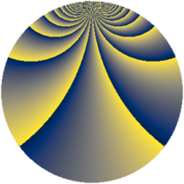Properties

 Label 1152.2.wLevel $1152$ Weight $2$ Character orbit 1152.w Rep. character $\chi_{1152}(143,\cdot)$ Character field $\Q(\zeta_{8})$ Dimension $64$ Newform subspaces $2$ Sturm bound $384$ Trace bound $11$

Related objects

Defining parameters

 Level: $$N$$ $$=$$ $$1152 = 2^{7} \cdot 3^{2}$$ Weight: $$k$$ $$=$$ $$2$$ Character orbit: $$[\chi]$$ $$=$$ 1152.w (of order $$8$$ and degree $$4$$) Character conductor: $$\operatorname{cond}(\chi)$$ $$=$$ $$96$$ Character field: $$\Q(\zeta_{8})$$ Newform subspaces: $$2$$ Sturm bound: $$384$$ Trace bound: $$11$$

Dimensions

The following table gives the dimensions of various subspaces of $$M_{2}(1152, [\chi])$$.

Total New Old
Modular forms 832 64 768
Cusp forms 704 64 640
Eisenstein series 128 0 128

Trace form

 $$64 q + O(q^{10})$$ $$64 q + 64 q^{55} + 64 q^{61} + 32 q^{67} + 64 q^{79} + 96 q^{91} + O(q^{100})$$

Decomposition of $$S_{2}^{\mathrm{new}}(1152, [\chi])$$ into newform subspaces

Label Dim. $$A$$ Field CM Traces $q$-expansion
$a_{2}$ $a_{3}$ $a_{5}$ $a_{7}$
1152.2.w.a $32$ $9.199$ None $$0$$ $$0$$ $$0$$ $$0$$
1152.2.w.b $32$ $9.199$ None $$0$$ $$0$$ $$0$$ $$0$$

Decomposition of $$S_{2}^{\mathrm{old}}(1152, [\chi])$$ into lower level spaces

$$S_{2}^{\mathrm{old}}(1152, [\chi]) \cong$$ $$S_{2}^{\mathrm{new}}(96, [\chi])$$$$^{\oplus 6}$$$$\oplus$$$$S_{2}^{\mathrm{new}}(288, [\chi])$$$$^{\oplus 3}$$$$\oplus$$$$S_{2}^{\mathrm{new}}(384, [\chi])$$$$^{\oplus 2}$$